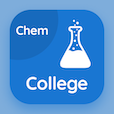Colleges Online Courses

College Chemistry Quizzes

College Chemistry Quiz PDF - Complete

# Charge to Mass Ratio of Electron Multiple Choice Questions p. 107

Study Charge to Mass Ratio of Electron multiple choice questions and answers, charge to mass ratio of electron quiz answers PDF 107 to study College Chemistry course online. Atomic Structure MCQ trivia questions, charge to mass ratio of electron Multiple Choice Questions (MCQ) for online college degrees. "Charge to Mass Ratio of Electron MCQ" PDF eBook: liquefaction of gases, photons wave number, classification of solids, electron distribution, charge to mass ratio of electron test prep for GRE prep classes.

"The e/m value of electron is measured for the certain mass of electrons whose minimum value is" MCQ PDF: 9 kg, 2 kg, 1 kg, and 6 kg for online degree programs. Learn atomic structure questions and answers to improve problem solving skills for best online ACT prep class.

## Charge to Mass Ratio of Electron Questions and Answers MCQs

MCQ: The e/m value of electron is measured for the certain mass of electrons whose minimum value is

2 kg
9 kg
1 kg
6 kg

MCQ: The n+l value of 3d orbital is

8
2
5
3

MCQ: The lattice energy of NaCl is

600 kilo joule per mole
787 kilo joule per mole
900 kilojoule per mole
500 kilo joule per,ole

MCQ: Spectral lines have wavelength and wave-number which depends upon the quantity of

energy emitted by electron
electrons
light
atoms

MCQ: The critical volume for hydrogen is

74.42cm cube
95.65 cm cube
64.51cm cube
89cm cube

### More Quizzes from College Chemistry Course